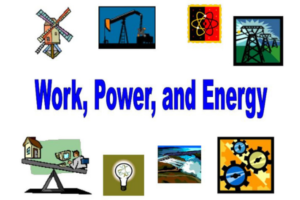Fri. Dec 1st, 2023

# Work Energy and Power: Let us understand the concept of SI units

## Understanding the Concept, Formulae&amp; S.I Units of Work Energy and Power

### Work

Work results when a force acts upon an object to cause a displacement (or a motion) or, in some instances, to hinder a motion. We can say that when constant force F, acts on a particle while it moves through a displacement S, then the force is said to do work W on the particle given by.Equation- W= F.S = FS cos

Where is the smaller angle between F and S.
Important points related to work-
• If the force and the displacement are in the same direction = 0 degree, therefore- W= FS
• If the force and displacement are perpendicular to each other, = 90 degree, then W= FScos90 degree.
• Work is a scalar quantity
• In SI system the absolute Standard Unit of Work is Joule whereas in CGS system the Unit of Work is Erg
• Work done by a constant force in moving from one point to another depends only on the initial and final positions and not on the actual path followed between two points.
• If the work done by a force during a round trip of a system is zero, the force is said to be conservative, otherwise it is called non-conservative. Force of friction is called non conservative force.

### Energy-

Energy can be defined as the capacity of the body for doing work, i.e. it is the total amount of work stored up in the body. Since energy of a body represents the total amount of work done, so it’s a scalar quantity.

### Important points related to Energy-

• Energy can exist in different forms such as mechanical energy, heat energy, sound energy, electrical energy, light energy etc.
• Mechanical energy is of two types- kinetic energy and potential energy.
• Kinetic energy of a body is further the energy acquired by the body by virtue of its motion. If the body of mass M moves with velocity V, its kinetic energy is given by KE=1/2 m
• Potential energy of a body is the energy possessed by the body by virtue of its position or configuration
• If a body of mass m be raised through a vertical height against gravity then its gravitational potential energy will be PE= mgh
• Work done by a force acting on a body is equal to change in kinetic energy of a body. This is called as work-energy theorem of the body.

### Power

A small engine can do just as much work as a larger engine, but it takes longer to do it. Whereas the larger engine can do work at a faster rate. So, we can say that the rate at which work is done is called Power.
Power= Work done/ Time taken
Or Power= Energy transferred/ time taken

Power is measured in Joules per sec (J/s). or watts (W) If a body does 1J of useful work every second, it has a power output of 1W. If a body does 4000 J of work in 10 s, it has a power output of 400W. Larger powers are also given in kilowatts or megawatts.

For more such informative articles stay tuned to OWN TV.